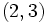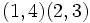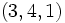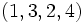# Full tetrahedral group is isomorphic to S4

Jump to: navigation, search
This article gives a proof/explanation of the equivalence of multiple definitions for the term symmetric group:S4
View a complete list of pages giving proofs of equivalence of definitions

## Statement

Consider the full tetrahedral group. This is the group of all self-isometries of$\R^3$ that send a particular regular tetrahedron to itself. The claim is that this group is isomorphic to symmetric group:S4.

## Proof

### Construction of the homomorphism

The regular tetrahedron has four vertices. Number these vertices 1,2,3,4.

Every element of the full tetrahedral group permutes the vertices of the regular tetrahedron among themselves. Moreover, composing elements of the group composes the actions on the vertices. Therefore, the full tetrahedral group has a group action on the set$\{1,2,3,4\}$. Therefore, there is a homomorphism of groups from the full tetrahedral group to symmetric group:S4 defined by this action.

### Injectivity

The kernel of the homomorphism comprises those isometries of$\R^3$ that fix all four vertices of the regular tetrahedron. We will show that this is trivial

Since the four vertices of a regular tetrahedron are affinely independent in the three-dimensional space$\R^3$, they affinely generate all of$\R^3$, i.e., every point of$\R^3$ is expressible as an affine linear combination of these. Thus, any affine linear automorphism of$\R^3$ that fixes these four points must be the identity map. Any self-isometry of$\R^3$ is an affine linear automorphism, so any slef-isometry of$\R^3$ that fixes all points is the identity map.

### Surjectivity

The essential argument is that the roles of the four points are completely interchangeable at the conceptual level, so every permutation of these must arise from a self-isometry of$\R^3$.

There are two more explicit styles of proof:

We will follow the former approach below, because it encompasses the latter and also provides more information about the group structure. Note that the even permutations are realized via orientation-preserving isometries (which in this case includes only rotations, due to Euler's theorem) and the odd permutations involve reflections.

Partition Partition in grouped form Verbal description of cycle type Elements with the cycle type Size of conjugacy class Even or odd? How it is obtained from an isometry
1 + 1 + 1 + 1 1 (4 times) four cycles of size one each, i.e., four fixed points$()$ -- the identity element 1 even The identity map on$\R^3$
2 + 1 + 1 2 (1 time), 1 (2 times) one transposition (cycle of size two), two fixed points$(1,2)$,$(1,3)$,$(1,4)$,$(2,3)$,$(2,4)$,$(3,4)$ 6 odd The reflection about the plane that is the perpendicular bisector of the line segment joining the two vertices that we wish to transpose.
2 + 2 2 (2 times) double transposition: two cycles of size two$(1,2)(3,4)$,$(1,3)(2,4)$,$(1,4)(2,3)$ 3 even The half-turn (rotation by angle of$\pi$) about the line joining the midpoints of the edges between the pairs of vertices we want to interchange. For instance, to achieve$(1,2)(3,4)$, we do a half-turn about the line joining (the midpoint between the vertices 1 and 2) and (the midpoint between the vertices 3 and 4).
3 + 1 3 (1 time), 1 (1 time) one 3-cycle, one fixed point$(1,2,3)$,$(1,3,2)$,$(2,3,4)$,$(2,4,3)$,$(3,4,1)$,$(3,1,4)$,$(4,1,2)$,$(4,2,1)$ 8 even Rotation by an angle$2\pi/3$ (clockwise or counter-clockwise, depending on the permutation we desire) about an axis through the center of and perpendicular to the plane of the triangle comprising the three vertices we want to cycle. This axis passes through the fourth vertex. For instance, to achieve the permutation$(1,2,3)$, rotate by$2\pi/3$ (in the appropriate direction) about the axis through the center of the triangle of vertices 1, 2, and 3, and perpendicular to the plane of the triangle.
4 4 (1 time) one 4-cycle, no fixed points$(1,2,3,4)$,$(1,2,4,3)$,$(1,3,2,4)$,$(1,3,4,2)$,$(1,4,2,3)$,$(1,4,3,2)$ 6 odd (complicated, we need to realize this by composing the explicitly described isometries for the other permutations)